• python实现岭回归
千次阅读
2019-05-07 22:52:37

# 岭回归实现（最小二乘法的带惩罚项版）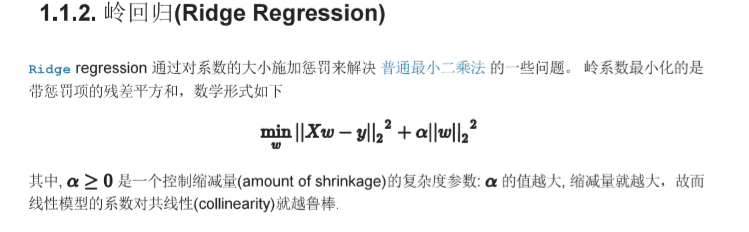# 代码实现

import numpy as np
import matplotlib.pyplot as plt
from sklearn import linear_model

def main():
# X 是一个 10x10 的 希尔伯特矩阵(Hilbert matrix)
X = 1. / (np.arange(1, 11) + np.arange(0, 10)[:, np.newaxis])
#十个1的向量
y = np.ones(10)

# 计算路径(Compute paths)
n_alphas = 200
alphas = np.logspace(-10, -2, n_alphas)

coefs = []
for a in alphas:
ridge = linear_model.Ridge(alpha=a, fit_intercept=False)    #每个循环都要重新实例化一个estimator对象
ridge.fit(X, y)
coefs.append(ridge.coef_)
# 展示结果
ax = plt.gca()
ax.plot(alphas, coefs)
ax.set_xscale('log')
ax.set_xlim(ax.get_xlim()[::-1])  # 反转数轴，越靠左边 alpha 越大，正则化也越厉害
plt.xlabel('alpha')
plt.ylabel('weights')
plt.title('Ridge coefficients as a function of the regularization')
plt.axis('tight')
plt.show()
if __name__='__main__':
main()


# 运行结果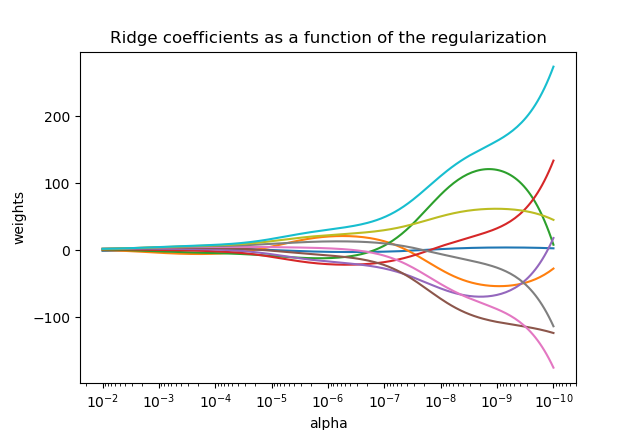更多相关内容
• 运用python编写的岭回归，封装成了class
• 机器学习中线性回归以及岭回归算法的实现，Python版本
• 代码功能介绍在《初探 岭回归 LASSO回归 （python 实现）》中有详细的介绍，文章中若有不正确的，也希望能够不吝赐教，相互学习。
• 用于岭回归python代码（已实现以预测下个月的CO2浓度） 资料可用性 文件 Ridge.py ：标准函数和Ridge回归函数window_make.py ：使用滑动窗口方法制作大小为p（窗口大小）的时间序列列表。 Final_version.ipynb ：...
• 写在最前面： 当数据的特征大于样本点，线性回归就不能...简单来说，岭回归就是在矩阵(X^T)*X的基础上加上λI，这样使得矩阵非奇异，从而能对(XTX)-1+λI整体求逆（矩阵X的转置乘矩阵X再求逆矩阵，实在是不会打数学...

写在最前面：
当数据的特征大于样本点，线性回归就不能用了，因为在计算[(X^T)*X]的逆时候，n>m，n是特征，m是样本点，此时的输入矩阵不是满秩矩阵，行列式为0。

此时，我们可以使用岭回归（ridge regression)

阅读本文前，需要各位简单回忆一下线性代数知识，关于矩阵的秩

简单来说，岭回归就是在矩阵(X^T)*X的基础上加上λI，这样使得矩阵非奇异，从而能对(XTX)-1+λI整体求逆（矩阵X的转置乘矩阵X再求逆矩阵，实在是不会打数学公式，相信大家都能看的懂哈)，其中，I是一个m✖️m的单位矩阵，这样我们的回归系数变成了下面上代码

截图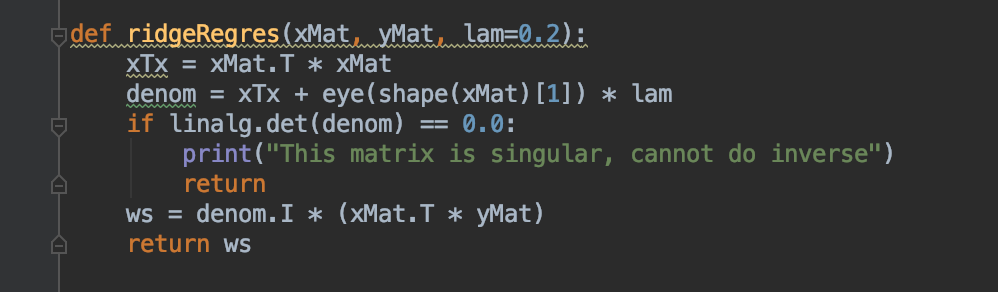这个函数首先构建矩阵X^T ✖️ X，然后用lam乘以单位矩阵，默认lam为0.2，此时不排除lam为0的情况，所以还是需要对行列式进行一下非0判断。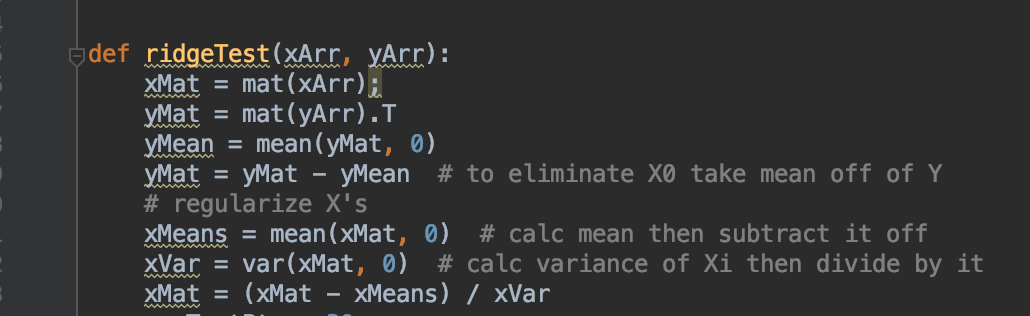上面是数据标准化
具体做法是所有特征减去各自的均值并除以方差
也可以计算标准差，std函数，标准差是方差开根号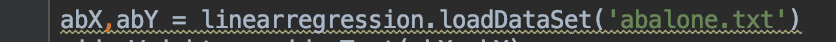加载数据

这样就得到了30个不同lambda对应的回归系数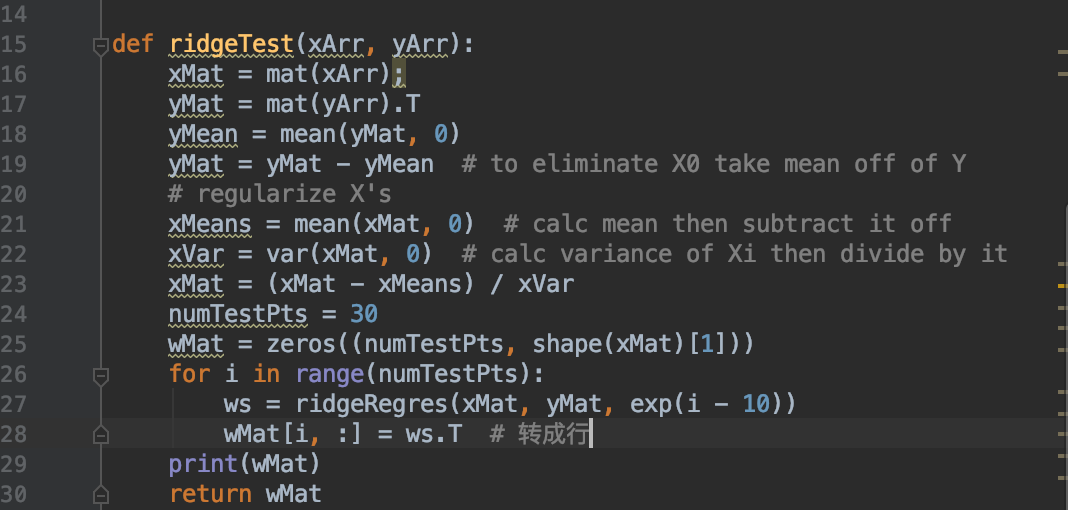简单介绍几个numpy常用的函数
mat：创建矩阵
mean：求均值
var：求方差
exp：指数

这里的lambda采用指数级变化，可以较快、和更明显的看出lambda在取值很小和很大的情况下，对结果造成的影响，将所有回归系数输出到一个矩阵并返回：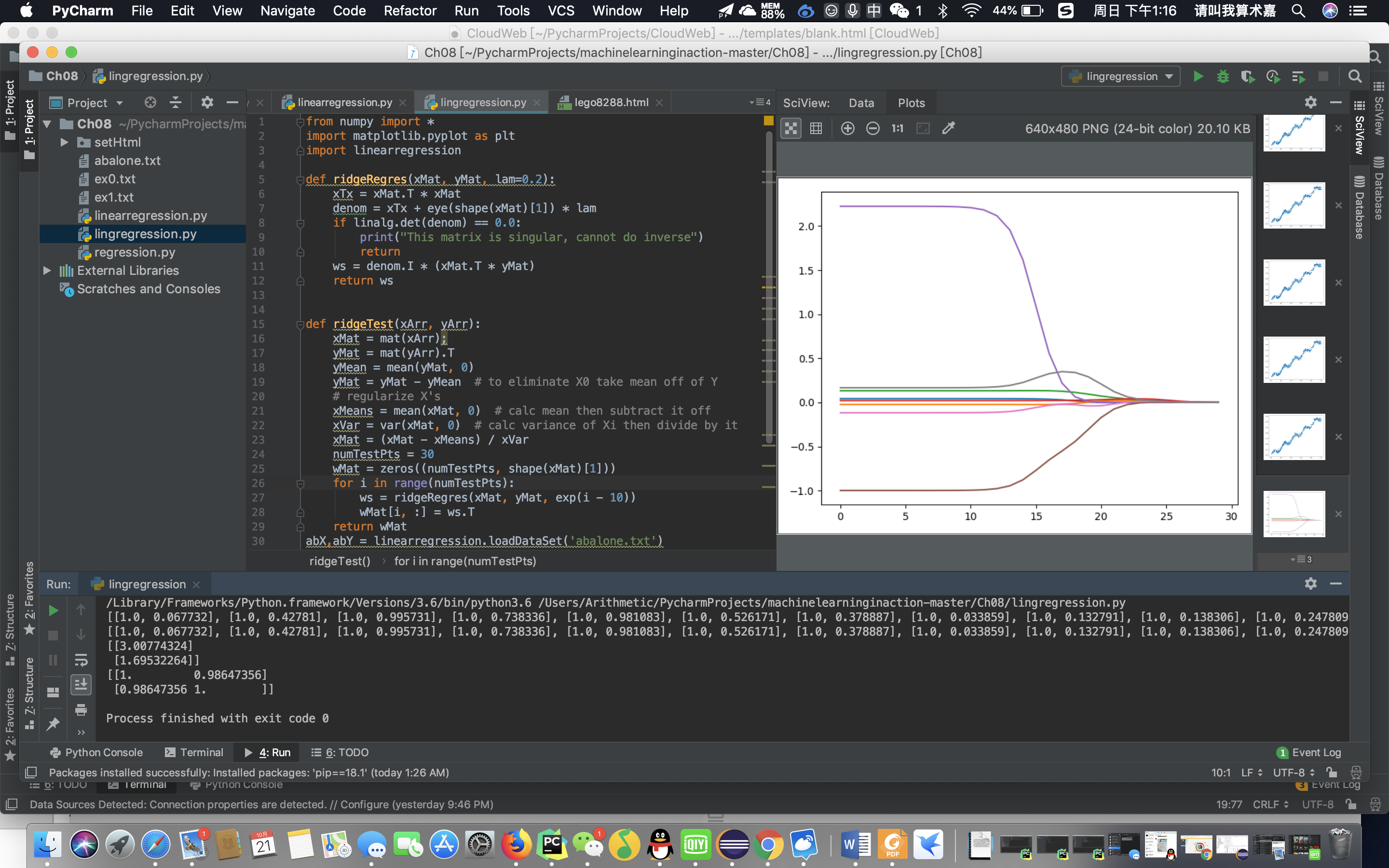横坐标为log(λ)，纵坐标为回归系数
当λ无限趋于0时，此时就是线性回归
当λ变大时，回归系数为0，此时也没有任何意义
在中间部分的某值可以取到比较好的预测效果

今天就到这里，好困啊，大家有啥想看的可以私我微信：18351922995

展开全文机器学习
• 常见的回归：线性回归、岭回归、非线性回归 回归拟合目标：计算自变量与因变量关系的函数参数 通过不断拟合缩小预测值与真实值的差距：最终使得这个差距（误差项）成为一组均值为0，方差为1的随机数。 2、损失函数 ...
• 一、标准线性回归   在线性回归中我们要求的参数为： 详细的推导可以参见：...所以代码实现主要就是实现上式，python代码如下： import numpy as np import pandas as pd import matplotlib.pyplo

# 一、标准线性回归

在线性回归中我们要求的参数为：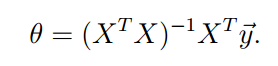所以代码实现主要就是实现上式，python代码如下：

import numpy as np
import pandas as pd
import matplotlib.pyplot as plt

# implement stand regress
def standRegress(xArr,yArr):
# 将数组转换为矩阵
xMat = np.mat(xArr)
yMat = np.mat(yArr)
xTx = xMat.T * xMat # 计算xTx的
if np.linalg.det(xTx) == 0.0:
print('xTx不能求逆矩阵')
return
theta = xTx.I * (xMat.T * yMat)
yHat = xMat*theta
return yHat

# import data

# deal with data
xArr = []
yArr = []
for data in ex0:
# print(data)
xTmp = []
yTmp = []
xTmp.append(data)
xTmp.append(data)
yTmp.append(data)
xArr.append(xTmp)
yArr.append(yTmp)

# print(ex0)
# print(xArr[0:2])
print(yArr)
# ws = standRegress(xArr,yArr)
# print(ws)
yHat = standRegress(xArr,yArr)
xMat = np.mat(xArr)
yMat = np.mat(yArr)
# print(yMat.T[0,:].flatten().A)
plt.scatter(xMat[:,1].flatten().A,yMat.T[0,:].flatten().A) # real data
plt.plot(xMat[:,1],yHat,'r-') # predict data
plt.show()


运行结果如下：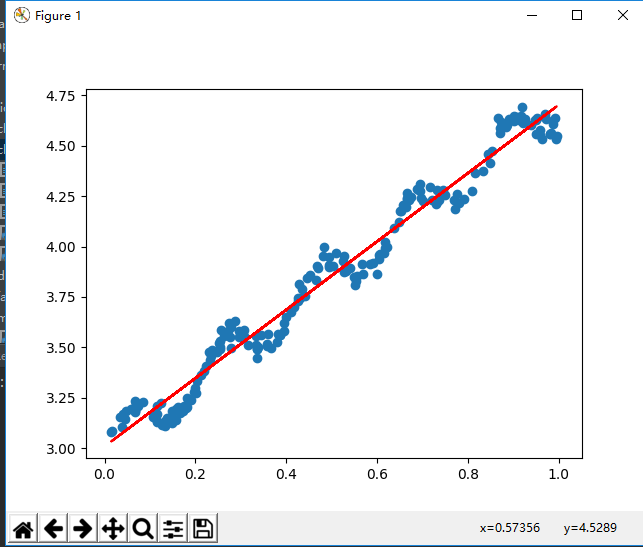# 二、局部加权线性回归

局部加权线性回归是在线性回归的基础上增加权值，以更好的拟合弯曲的线段（详细参见：http://blog.csdn.net/weiyongle1996/article/details/74537567

使用局部加权解出的回归系数为：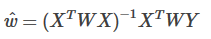python代码如下：

import numpy as np
import matplotlib.pyplot as plt

def lwlr(testPoint,xArr,yArr,k=1.0):
xMat = np.mat(xArr)
yMat = np.mat(yArr)
m = np.shape(xMat) #shape 读取矩阵的长度 shape获得矩阵第一维的长度
# print(m)
weights = np.mat(np.eye(m)) # 创建对角矩阵
# print(weights)
for j in range(m):                      #next 2 lines create weights matrix
diffMat = testPoint - xMat[j,:]     #矩阵每行的差
weights[j,j] = np.exp(diffMat*diffMat.T/(-2.0*k**2)) # 计算权重
xTx = xMat.T * (weights * xMat)
if np.linalg.det(xTx) == 0.0:
print("This matrix is singular, cannot do inverse")
return
ws = xTx.I * (xMat.T * (weights * yMat))
return testPoint * ws

def lwlrTest(testArr,xArr,yArr,k=1.0):
m = np.shape(testArr)
yHat = np.zeros(m)
for i in range(m):
yHat[i] = lwlr(testArr[i],xArr,yArr,k)
return yHat

# import data

# deal with data
xArr = []
yArr = []
for data in ex0:
# print(data)
xTmp = []
yTmp = []
xTmp.append(data)
xTmp.append(data)
yTmp.append(data)
xArr.append(xTmp)
yArr.append(yTmp)

# 对单点估计
# yHat = lwlr(xArr,xArr,yArr,1.0)
# print(yHat)
# 得到所有点的估计
yHat = lwlrTest(xArr,xArr,yArr,0.02)
xMat = np.mat(xArr)
yMat = np.mat(yArr)
# print(xMat)
strInd = xMat[:,1].argsort(0) # argsort返回数组值从小到大排列后各元素对应的索引值
# print(strInd)
xSort = xMat[strInd][:,0,:] # 排序
# print(xSort)

plt.scatter(xMat[:,1].flatten().A,yMat.T[0,:].flatten().A) # real data
plt.plot(xSort[:,1],yHat[strInd],'r-') # predict data
plt.show()

运行结果如下：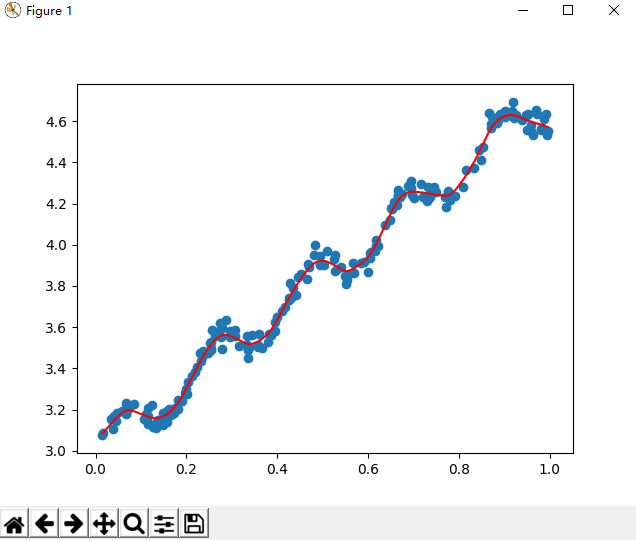更改k的值会获得不同的曲线，k越小，对真实数据拟合的越好（但可能过拟合），k越大，越趋向于标准的线性回归。

# 三、岭回归

岭回归就是在矩阵xTx上增加一项使得矩阵非奇异，从而能够对其求逆。从上面两端代码我们可以看到，在之前对xTx求逆时都需要先判断xTx是否可以求逆，而岭回归就是解决这个问题的。岭回归的回归系数计算公式为：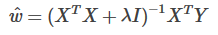实现代码如下：

import numpy as np
import matplotlib.pyplot as plt

def ridgeRegres(xMat,yMat,lam=0.2):
xTx = xMat.T*xMat
denom = xTx + np.eye(np.shape(xMat))*lam
if np.linalg.det(denom) == 0.0:
print("This matrix is singular, cannot do inverse")
return
ws = denom.I * (xMat.T*yMat)
return ws

def ridgeTest(xArr,yArr):
xMat = np.mat(xArr); yMat=np.mat(yArr).T
yMean = np.mean(yMat) # 数据标准化
# print(yMean)
yMat = yMat - yMean
# print(xMat)
#regularize X's
xMeans = np.mean(xMat,0)
xVar = np.var(xMat,0)
xMat = (xMat - xMeans) / xVar #（特征-均值）/方差
numTestPts = 30
wMat = np.zeros((numTestPts,np.shape(xMat)))
for i in range(numTestPts): # 测试不同的lambda取值，获得系数
ws = ridgeRegres(xMat,yMat,np.exp(i-10))
wMat[i,:]=ws.T
return wMat

# import data
xArr = ex0[:,0:-1]
yArr = ex0[:,-1]
# print(xArr,yArr)
ridgeWeights = ridgeTest(xArr,yArr)
# print(ridgeWeights)
plt.plot(ridgeWeights)
plt.show()
运行结果如图：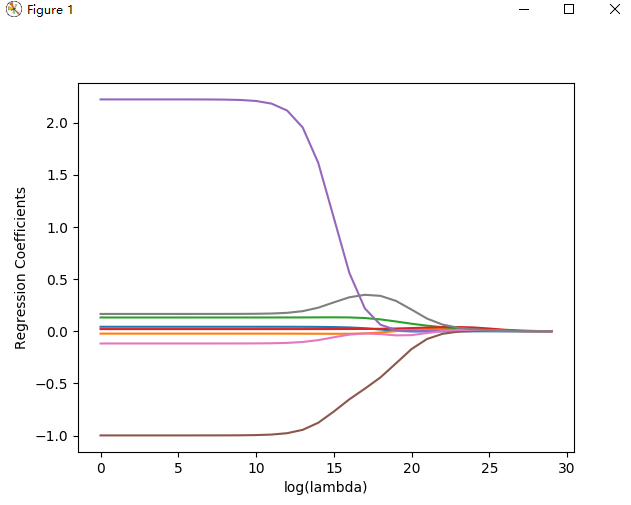纵坐标为回归系数，横坐标为log(lambda)，在最左边，回归系数与线性回归一致，最右边系数全部缩减为0.

其中间某部分可以得到最好的预测结果，为了定量进行寻找最佳参数，还需要进行交叉验证。

以上代码python环境均为python3.6

代码参考：

《机器学习实战》

数据取自《机器学习实战》附带数据

展开全文• 1.2 岭回归 1.3 过拟合 2sklearn中的岭回归 3 案例 3.1 数据介绍： 3.2 实验目的： 3.3 数据特征如下： 4 Python实现 4.1 代码 4.2 结果 5 正则化 1 概述 1.1 线性回归 对于一般地线性回归问题，...

目录

1 概述

1.1 线性回归

1.2 岭回归

1.3 过拟合

2 sklearn中的岭回归

3 案例

3.1 数据介绍：

3.2 实验目的：

3.3 数据特征如下：

4 Python实现

4.1 代码

4.2 结果

5 正则化

## 1 概述

### 1.1 线性回归

对于一般地线性回归问题，参数的求解采用的是最小二乘法，其目标函数如下：

参数 w 的求解，也可以使用如下矩阵方法进行：

这个公式看着吓人，其实推导过程简单由（ 推导而来，纸老虎）
对于矩阵 X ，若某些列线性相关性较大（即训练样本中某些属性线性相关），就会导致
的值接近 0 ，在计算 时就会出现不稳定性。
结论 传统的基于最小二乘的线性回归法缺乏稳定性。

### 1.2 岭回归

岭回归的优化目标：

对应的矩阵求解方法为：

岭回归(ridge regression) 是一种专用于共线性数据分析的有偏估计回归方法。
是一种改良的最小二乘估计法，对某些数据的拟合要强于最小二乘法。

### 1.3 过拟合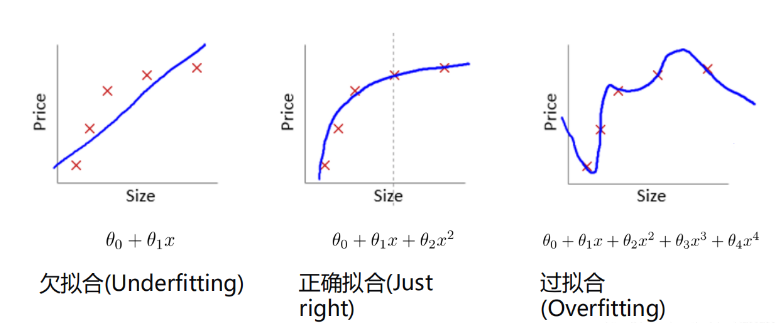图二就是正常拟合，符合数据的趋势，而图三，虽然在训练集上拟合得很好，但是出现未知数据时，比如Size很大时，根据目前拟合来看，可能得到的结果很小，与实际误差会很大。

## 2 sklearn中的岭回归

在sklearn库中，可以使用sklearn.linear_model.Ridge调用岭回归模型，其主要参数有：
alpha：正则化因子，对应于损失函数中的 𝜶
fit_intercept：表示是否计算截距，
solver：设置计算参数的方法，可选参数‘auto’、‘svd’、‘sag’等。

## 3 案例

交通流量预测实例：

### 3.1 数据介绍：

数据为某路口的交通流量监测数据，记录全年小时级别的车流量。

### 3.2 实验目的：

根据已有的数据创建多项式特征，使用岭回归模型代替一般的线性模型，对 车流量 的信息进行 多项式回归

### 3.3 数据特征如下：

HR ：一天中的第几个小时（0-23）
WEEK_DAY ：一周中的第几天（0-6）
DAY_OF_YEAR ：一年中的第几天（1-365）
WEEK_OF_YEAR ：一年中的第几周（1-53）
TRAFFIC_COUNT ：交通流量
全部数据集包含2万条以上数据（21626）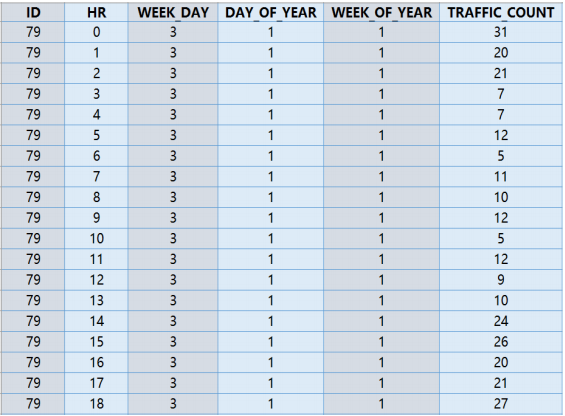## 4 Python实现

### 4.1 代码

#*================1. 建立工程，导入sklearn相关工具包====================**
import numpy as np
from sklearn.linear_model import Ridge   #通过sklearn.linermodel加载岭回归方法
from sklearn import model_selection     #加载交叉验证模块
import matplotlib.pyplot as plt      #加载matplotilib模块
from sklearn.preprocessing import PolynomialFeatures     #通过加载用于创建多项式特征，如ab、a2、b2

#*=================2. 数据加载=========================================**
data=np.genfromtxt('岭回归.csv',delimiter=',')     #使用numpy的方法从csv文件中加载数据
print(data)
print(data.shape)
plt.plot(data[:,4])                #使用plt展示车流量信息
#plt.show()
#*================3. 数据处理==========================================**
X=data[:,:4]                  #X用于保存0-3维数据，即属性
y=data[:,4]                   ##y用于保存第4维数据，即车流量
poly=PolynomialFeatures(6)    #用于创建最高次数6次方的的多项式特征，多次试验后决定采用6次
X=poly.fit_transform(X)       #X为创建的多项式特征

#*================4. 划分训练集和测试集=================================**
train_set_x, test_set_x , train_set_y, test_set_y =model_selection.train_test_split(X,y,test_size=0.3,
random_state=0)
#将所有数据划分为训练集和测试集，test_size表示测试集的比例，
# #random_state是随机数种子

#*==============5. 创建回归器，并进行训练===============================**
clf=Ridge(alpha=1.0,fit_intercept = True)
#接下来我们创建岭回归实例
clf.fit(train_set_x,train_set_y) #调用fit函数使用训练集训练回归器
clf.score(test_set_x,test_set_y) #利用测试集计算回归曲线的拟合优度，clf.score返回值为0.7375
#拟合优度，用于评价拟合好坏，最大为1，无最小值，当对所有输入都输出同一个值时，拟合优度为0。

#*============6. 画出拟合曲线=========================================**
start=100                      #接下来我们画一段200到300范围内的拟合曲线
end=200
y_pre=clf.predict(X)           #是调用predict函数的拟合值
time=np.arange(start,end)
plt.plot(time,y[start:end],'b', label="real")
plt.plot(time,y_pre[start:end],'r', label='predict')   #展示真实数据（蓝色）以及拟合的曲线（红色）
plt.legend(loc='upper left') #设置图例的位置
plt.show()



### 4.2 结果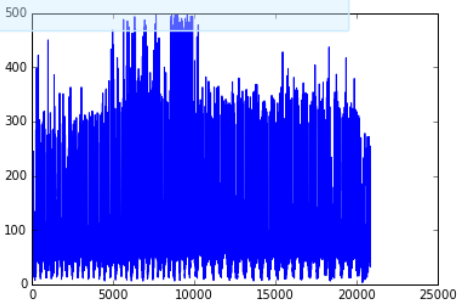分析结论 ：预测值和实际值的走势大致相同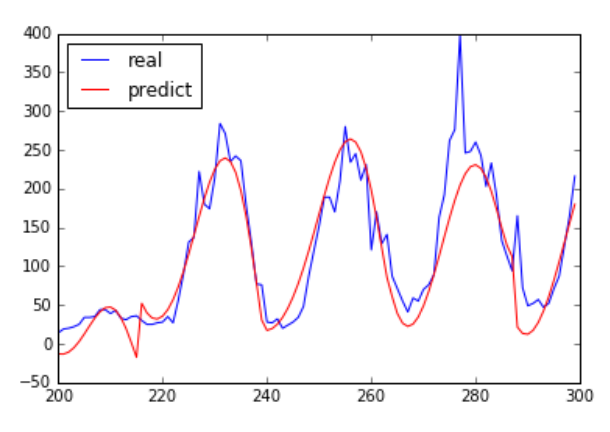## 5 正则化

原理解析-过拟合与正则化

展开全文人工智能
• 岭回归的原理：首先要了解最小二乘法的回归原理设有多重线性回归模型 y=Xβ+ε ,参数β的最小二乘估计为当自变量间存在多重共线性,|X‘X|≈0时，设想|X‘X|给加上一个正常数矩阵(k>0)那么|X‘X|+kI 接近奇异的...
• ## Python实现岭回归

千次阅读 2018-09-25 13:23:07
题目： ...在每组数据集上用具有不同的7阶多项式进行岭回归拟合 import numpy as np import matplotlib.pyplot as plt from tkinter import _flatten x_arange = 0.041 * np.arange(0, 25, 1) #每...
• [机器学习-5]岭回归[L2正则化]及python实现（Ridge Regression）【L2】前言题目岭回归（Ridge Regression）k-fold validation(1)实现（realization）(2)实现 前言 ！！实现直接跳转实现 这章本来是为了接前面的过...
• 岭回归，又叫吉洪诺夫正则化，是由Hoerl和Kennard于1970年提出的是一种专用于共线性数据分析的有偏估计回归法。岭回归实际上是一种改良的最小二乘估计法，具有L2正则化的线性最小二乘法。回归算法的，本质就是为了...机器学习 人工智能 算法
• 岭回归与多项式回归的最大区别就是损失函数上的区别。岭回归的代价函数如下：为了方便计算导数，通常也会写成以下形式：上述式子中w为长度为n的向量，不包括偏置项的系数 θ0，θ是长度为n+1的向量，包括偏置项系数...
• 作为一名曾经研究过人工神经网络的菜鸟，前两天刚听说有岭回归估计和LASSO估计，统计学老师也布置了作业，然，在不甚理解的情况下，用Python写了一下，也不知是否正确。不合适的地方请不吝赐教。 作业如下： x，...岭估计
• 原标题：Python 机器学习算法实践：岭回归和LASSO来源：伯乐在线 - iPytLab前言继续线性回归的总结, 本文主要介绍两种线性回归的缩减(shrinkage)方法的基础知识: 岭回归(Ridge Regression)和LASSO(Least Absolute ...
• 接着上节讨论，岭回归也是一种用于回归的线性模型，因此它的预测公式与普通的最小二乘法相同。但在岭回归中，对系数(w)的选择不仅要在训练数据上得到好的预测结果，而且要拟合附加约束。同时我们还希望系数尽量小(w...
• python代码 岭回归 机器学习实战 岭回归计算公式 下面是python代码 # 岭回归 import numpy as np import os os.chdir('...\\MachineLearning\\Regression') # 设置路径 # 创建数据集 def ...
• 1、回归分析概括。 2、损失函数。 3、优化算法。 4、python的API。 5、python机器学习线性模型API。 6、机器学习中回归性能评估。 7、欠拟合和过拟合。...8、线性回归的改进--岭回归。 9、案例代码回归分析 损失函数 线性模型
• 1 岭回归对于一般地线性回归问题，参数的求解采用的是最小二乘法，其目标函数如下： argmin||Xw−y||2argmin || Xw-y ||^2 参数w的求解，也可以使用如下矩阵方法进行： w=(XTX)−1XTyw=(X^TX)^{-1}X^Ty 对于矩阵...机器学习
• 多元回归分析(Multiple Regression Analysis)是指在相关变量中将一个变量视为因变量，其他一个或多个变量视为自变量，建立多个变量之间线性或非线性数学模型数量关系式并利用样本数据进行分析的统计分析方法。...
• 1.岭回归 今天看了会L2正则化，大致的意思就是在惩戒函数中加入高次项的惩戒项，用来减小线性回归中高次项的系数，避免过拟合的状态。这样就产生了一种具有正则化的线性回归模型------岭回归。 2.岭回归在sklearn中...机器学习
•  当使用最小二乘法计算线性回归模型参数的时候，如果数据集合矩阵存在多重共...为了解决这个问题，就有了优化算法 岭回归（Ridge Regression ）。   多重共线性 在介绍岭回归之前时，先了解一下多重共线性。...机器学习
• 1 #-*- coding: utf-8 -*-2 """3 Created on Wed Oct 17 21:14:44 201845 @author: Luove6 """7 #KRR适合分类和回归训练集很少时，非线性方法8 importos9 importnumpy as np10 importmatplotlib.pyplot as plt11 ...
• 在介绍岭回归算法与Lasso回归算法之前，先要回顾一下线性回归算法。根据线性回归模型的参数估计公式可知可知，得到的前提是矩阵可逆。换句话说就是样本各个特征(自变量)之间线性无关。然而在实际问题中，常常会出现...
• 根据statamodels的OLS.fit_regularized()方法做岭回归，但是报错NotImplementedError。请问是什么原因？ 另外请问NotImplementedError这个error具体是什么意思？百度的结果大概是功能没实现，但这里官方api是有的...
• 这里主要讨论下广义回归中的经典算法~局部加权线性回归和岭回归以及如何用Python代码实现算法。内容上虽然不是学术论文，但同样遵循学术的严谨性，因此可能会有部分数理知识。本人也很讨厌成篇的理论推导，不过在...局部加权线性回归 算法
• 使用python实现多元线性回归，内容包含数据源及代码实现...